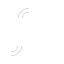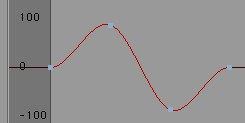ShapesRidge

Creates a ridge-shaped curve consisting of four points. The shape originates from the two center points and will lie at a position of 100 by default. The corresponding track must be selected.Ramp upRamp down

Creates a curve that ascends and descends linearly from 0 to 100 between the first and last key of a given track. The corresponding track must be selected.Formula...

Selecting this option will open a small dialog window in which you can enter simple or complex mathematical functions with which just about any imaginable F-Curve can be created. The Keys setting defines the number of in-betweens. The variable is t, which runs from 0 (sequence start) to 1 (sequence end). Angles for trigonometric functions must be given in radians. The corresponding track must be selected.

A llst with possible formulas can be found here.

The function arguments must always be placed in parenthesis. The number of open parenthesis must equal the number of close parenthesis (e.g., sin(sqr(exp(pi))).

# Example:

If you enter sin(t*2*pi) for s(t) and 4 for Keys, the following curve will result: